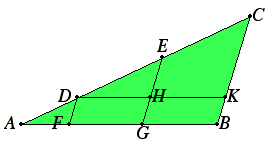# Proposition 10

To cut a given uncut straight line similarly to a given cut straight line.

Let AB be the given uncut straight line, and AC the straight line cut at the points D and E, and let them be so placed as to contain any angle.I.31

Join CB, and draw DF and EG through D and E parallel to CB, and draw DHK through D parallel to AB.

I.34

Therefore each of the figures FH and HB is a parallelogram. Therefore DH equals FG and HK equals GB.

VI.2

Now, since the straight line EH is parallel to a side CK of the triangle DCK, therefore, proportionally, DE is to EC as DH is to HK.

V.7

But DH equals FG, and HK equals GB, therefore DE is to EC as FG is to GB.

VI.2

Again, since DF is parallel to a side EG of the triangle AEG, therefore, proportionally, AD is to DE as AF is to FG.

But it was also proved that DE is to EC as FG is to GB, therefore DE is to EC as FG is to GB, and AD is to DE as AF is to FG.

Therefore the given uncut straight line AB has been cut similarly to the given cut straight line AC.

Q.E.F.

## Guide

In a sense, this proposition is a generalization of the last one VI.9. Prop. VI.9 cut a line into two parts whose ratio was a given numerical ratio. This proposition cuts a line into two parts whose ratio is a given ratio of two other lines. Both propositions rely on VI.2 as a basis to make any conclusion about the ratio of two lines.

#### Use of this construction

This proposition is not used later in the Elements, but it is a basic construction of geometry.